# Prime Implicant chart for minimizing Cyclic Boolean functions

Prerequisite – K-Map (Karnaugh Map), Implicants in K-Map
A functions is said to be a Cyclic Boolean Function if there is no Essential prime Implicant in its respective K-Map.

Properties of Cyclic functions:

• Every prime Implicant is of same size.
• Every minterm is covered by at least two prime Implicants (which means no essential prime Implicants).
• Having no essential prime Implicants means that there exist more than one minimized Solution / Expression for such functions, which will further be realized using digital circuits.
• For a cyclic function we can have two minimal forms with no overlapping of prime Implicants.

Example:
Find the minimal expression for the following function.

`f(w, x, y, z) =(0, 2, 4, 5, 10, 11, 13, 15) `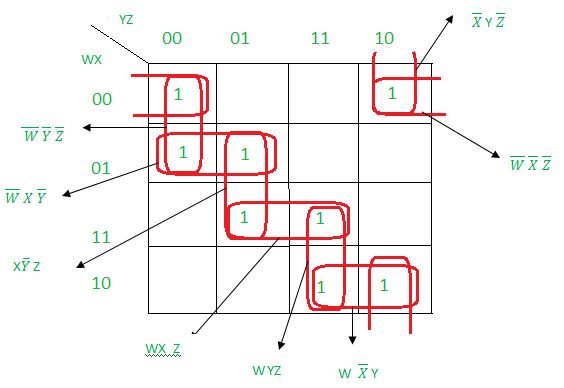As we can see in the above K-Map that there exist no essential prime Implicants. Here we can use prime Implicant chart to solve it easily.

Steps to solve above function using prime Implicant chart:

• Step-1:
Draw prime Implicant chart as below.The horizontal entries denote the given minterms which are mapped against all prime Implicants (vertically).The square boxes are crossed (‘x’) whenever a prime Implicant covers a perticular minterm in K-Map.

For example ‘WXZ’ prime Implicant is covering 13 and 15 therefore the corresponding squares are crossed(denoted by ‘x’).

Note that, A, B, C, D .., are variables used to denote all the prime Implicants.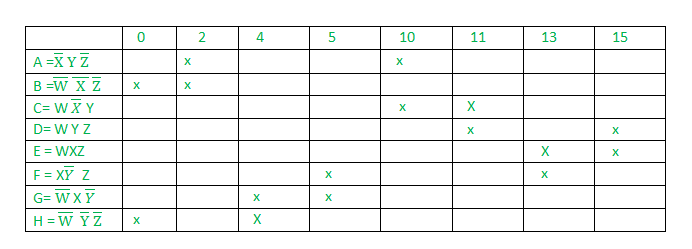• Step-2:
Arbitrarily choose any prime Implicant; check () the prime Implicant and the corresponding covered minterms as well. Now delete the row of the prime Implicant and corresponding columns of its minterms.

In our example prime Implicant A is chosen which is covering minterms 2 and 10. Therefor delete the row of A and the columns of 2 and 10. The arbitrarily chosen Prime Implicant (in our example A) must be present in the final minimal expression.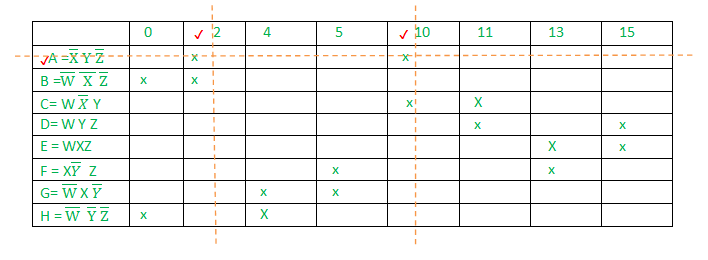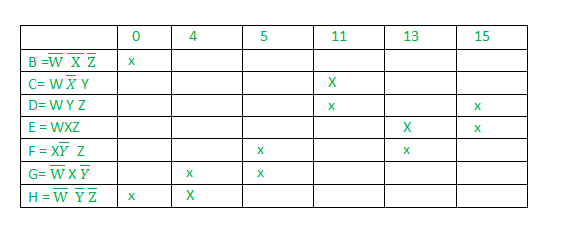• Step-3:
Find all such prime Implicant which are being covered by other prime Implicant completely and remove their corresponding rows (since these are non essential prime Implicants.).

In our example H is covering {0, 4} and B is covering {0} it means H is covering all the minterms that are covered by B, so delete B. Similarly D is covering C completely, so delete C.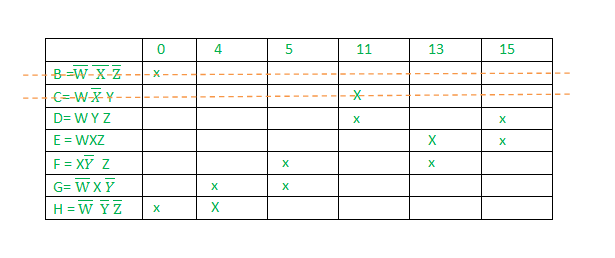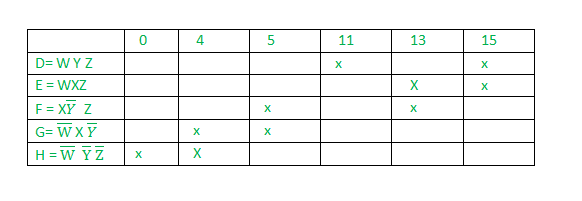• Step-4:
Now follow the standard procedure of prime Implicant chart mentioned in the sub steps given below:

1. Find the minterm which is covered by only one prime Implicant.
2. Check () that minterm, its corresponding prime Implicant and all other minterms which are covered by that corresponding prime Implicant.
3. If all the minterms are checked () stop the procedure, otherwise goto sub-step-1.

H is checked since 0 is covered by this prime Implicant only. We have also checked all other minterms which are covered by H (0 and 4). D is checked since 11 is covered by this prime Implicant only. We have also checked all other minterms which are covered by G (11 and 15).

Now the remaining minterms 5 and 13 are covered by F, therefore this prime Implicant is also checked.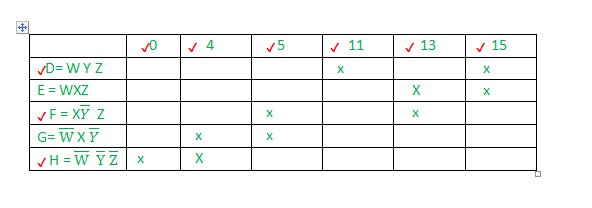Since no other minterm is left which is unchecked so stop the procedure. Now our minimal expression will contain all those prime Implicants which are checked() and the prime Implicant which was Arbitrarily deleted in step 2.

`Minimal Expression: A + D + F + H `

Note that Step-4 is self sufficient to get the minimal expression for all non-cyclic fucntions. Hence can be used as an alternative to K-Map (Especially when the function contains more than 4 variables).

Example-2: find the minimal expression for the following cyclic function.

`f(x, y, z) =(0, 1, 2, 5, 6, 7) `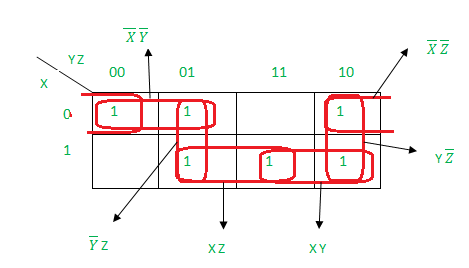Step-1:
Draw prime Implicant chart.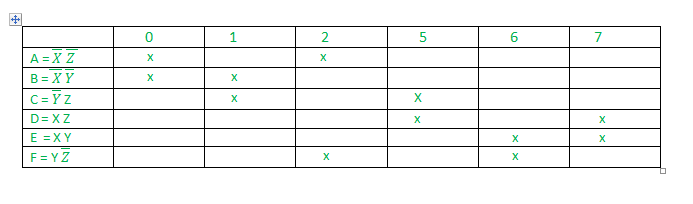Step-2:
A is chosen arbitrarily.Now the row of A and the columns of the corresponding minterms (0 and 2) are deleted.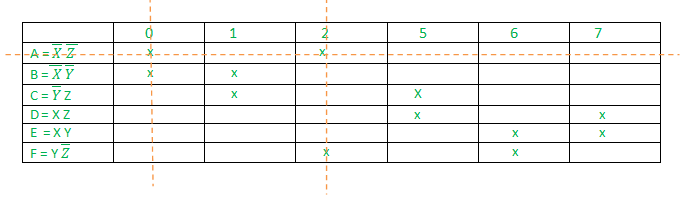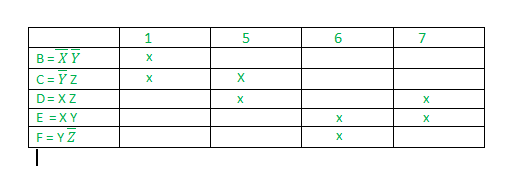Step-3:
Since B and F are completely covered by C and E respectively hence deleting prime Implicants B and F .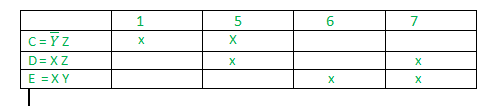Step-4:
Now follow the standard procedure of prime Implicant chart mentioned in Example-1.

Minterm 1 is covered by prime Implicant C only therefore check () C along with all the minterms which are covered by it (1 and 5).

Minterm 6 is covered by prime Implicant E only therefore check () E along with all the minterms which are covered by it (6 and 7).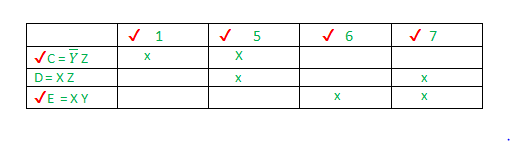Now since all the min terms (1, 5, 6, 7) are checked() therefore stop the procedure.

`Final Minimal Expression: A + C + E  `

Attention reader! Don’t stop learning now. Get hold of all the important CS Theory concepts for SDE interviews with the CS Theory Course at a student-friendly price and become industry ready.

My Personal Notes arrow_drop_upCheck out this Author's contributed articles.

If you like GeeksforGeeks and would like to contribute, you can also write an article using contribute.geeksforgeeks.org or mail your article to contribute@geeksforgeeks.org. See your article appearing on the GeeksforGeeks main page and help other Geeks.

Please Improve this article if you find anything incorrect by clicking on the "Improve Article" button below.

Article Tags :

3

Please write to us at contribute@geeksforgeeks.org to report any issue with the above content.